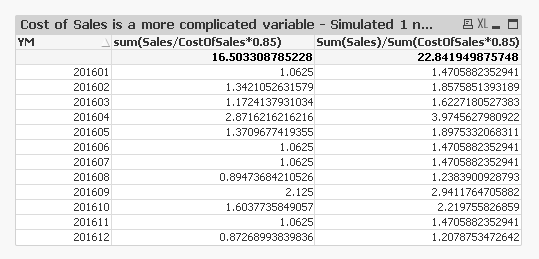# QlikView App Dev

Discussion Board for collaboration related to QlikView App Development.

Announcements
Our next Qlik Insider session will cover new key capabilities. Join us August 11th REGISTER TODAY
cancel
Showing results for
Did you mean:Creator II

## Alternatives to using relative %

Hi There,

I'm trying to develop alternatives to using a relative percentage where the expression is more complicated than e.g. sum(sales) which could then be done as sum(Sales).  I've attached some scenarios where I'm trying to calculate the relative % of sum(sales)/sum(cost of sales) and match this to the values generated by Qlikview's relative %.  The solutions I've come up with thus far though are a little complicated and some require aggr which I'd prefer to avoid.

My "Simulated 1" in the attached is the most simple

(sum(Sales)/sum(CostOfSales)

/

sum(Total Sales / CostOfSales))

But it seems to become difficult to maintain if using a more complicated variable instead of the field (as shown in second chart).  Is there some simple way of doing this that I'm missing?2 RepliesMVP

The problem is that there is a difference between

Sum(Sales)/Sum(\$(vCostOfSales))

and

Sum(Sales/\$(vCostOfSales))\

See how there value differ. So when you use this

Sum(TOTAL Sales/\$(vCostOfSales))

The denominator = 16.5033 vs. 22.84 you are looking to get....

The best way is to use your second alternative here....

(sum(Sales)/sum(\$(vCostOfSales)))

/

(sum(Total aggr(sum(Sales)/sum(\$(vCostOfSales)),YM)))Creator II
Author

Hi Sunny,

Thanks for the response.  I've gone with the second alternative in my charts.  This is actually for a Qliksense implementation which, very frustratingly, doesn't have an option for relative percentage.  The performance doesn't seem too bad at least.  Thanks for the advice.

RegardsCommunity Browser